# Inverse Matrix

Before calculating the inverse of a matrix let us understand what a matrix is? A matrix is a definite collection of objects arranged in rows and columns These objects are called elements of the matrix. The order of a matrix is written as number rows by number of columns. For example, 2 × 2, 2 × 3, 3 × 2, 3 × 3, 4 × 4 and so on. We can find the matrix inverse only for square matrices, whose number of rows and columns are equal such as 2 × 2, 3 × 3, etc. In simple words, inverse matrix is obtained by dividing the adjugate of the given matrix by the determinant of the given matrix. In this article, you will learn what a matrix inverse is, how to find the inverse of a matrix using different methods, properties of inverse matrix and examples in detail.

## Matrix Inverse

If A is a non-singular square matrix, there is an existence of n x n matrix A-1, which is called the inverse matrix of A such that it satisfies the property:

AA-1 = A-1A = I, where I is  the Identity matrix

The identity matrix for the 2 x 2 matrix is given by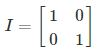Learn: Identity matrix

It is noted that in order to find the inverse matrix, the square matrix should be non-singular whose determinant value does not equals to zero.

Let us take the square matrix A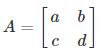Where a, b, c, and d represents the number.

The determinant of the matrix A is written as ad-bc, where the value of determinant should not equal to zero for the existence of inverse. The inverse matrix can be found for 2× 2, 3× 3, …n × n matrices. Finding the inverse of a 3×3 matrix is a bit more difficult than finding the inverses of a 2 ×2 matrix.

## Inverse Matrix Method

The inverse of a matrix  can be found using the three different methods. However, any of these three methods will produce the same result.

### Method 1: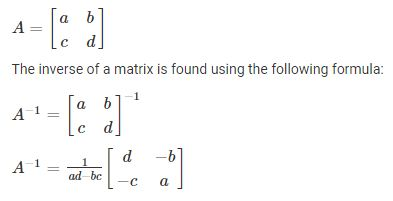Similarly, we can find the inverse of a 3×3 matrix by finding the determinant value of the given matrix.

Check out: Inverse matrix calculator

### Method 2:

One of the most important methods of finding the matrix inverse involves finding the minors and cofactors of elements of the given matrix. Observe the below steps to understand this method clearly.

• The inverse matrix is also found using the following equation:

where adj(A) refers to the adjoint of a matrix A, det(A) refers to the determinant of a matrix A.

• The adjoint of a matrix A or adj(A) can be found using the following method.

In order to find the adjoint of a matrix A first, find the cofactor matrix of a given matrix and then

take the transpose of a cofactor matrix.

• The cofactor of a matrix can be obtained as

Cij = (-1)i+j det (Mij)

Here, Mij refers to the (i,j)th minor matrix after removing the ith row and the jth column. You can also say that the transpose of a cofactor matrix is also called the adjoint of a matrix A.

Learn how to find the adjoint of a matrix here.

Similarly, we can also find the inverse of a 3 x 3 matrix. Here also the first step would be to find the determinant, followed by the next step – Transpose.

### Method 3:

Finding an Inverse Matrix by Elementary Transformation

Let us consider three matrices X, A and B such that X = AB. To determine the inverse of a matrix using elementary transformation, we convert the given matrix into an identity matrix. Learn more about  how to do elementary transformations of matrices here.

If the inverse of matrix A, A-1 exists then to determine A-1 using elementary row operations

1. Write A = IA, where I is the identity matrix of the same order as A.
2. Apply a sequence of row operations till we get an identity matrix on the LHS and use the same elementary operations on the RHS to get I = BA. The matrix B on the RHS is the inverse of matrix A.
3. To find the inverse of A using column operations, write A = IA and apply column operations sequentially till I = AB is obtained, where B is the inverse matrix of A.

Click here to understand the method of finding the inverse of a matrix using elementary operations.

### Inverse of a Matrix Formula

Let

$$\begin{array}{l}A=\begin{bmatrix} a &b \\ c & d \end{bmatrix}\end{array}$$
be the 2 x 2 matrix. The inverse matrix of A is given by the formula,

$$\begin{array}{l}A^{-1}=\frac{1}{ad-bc}\begin{bmatrix} d &-b \\ -c & a \end{bmatrix}\end{array}$$

Let

$$\begin{array}{l}A=\begin{bmatrix} a_{11} &a_{12} & a_{13}\\ a_{21} &a_{22} &a_{23} \\ a_{31} & a_{32} & a_{33} \end{bmatrix}\end{array}$$
be the 3 x 3 matrix. The inverse matrix is: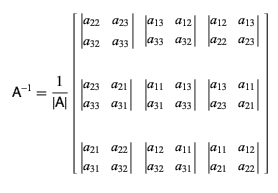### Inverse Matrix 2 x 2 Example

To understand this concept better let us take a look at the following example.

Example: Find the inverse of matrix A given below: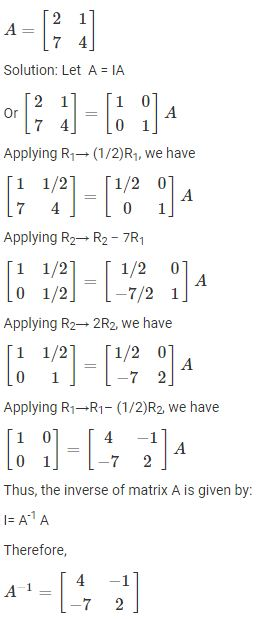### Inverse Matrix 3 x 3 Example

Problem: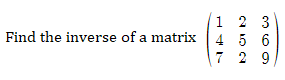Solution:

Determinant of the given matrix is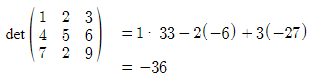Let us find the minors of the given matrix as given below: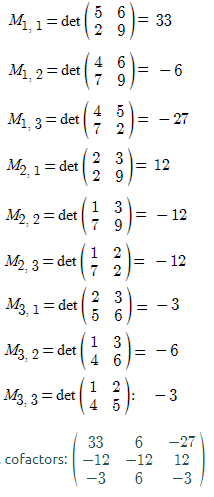Now, find the adjoint of a matrix by taking the transpose of cofactors of the given matrix.Now,

Hence, the inverse of the given matrix is: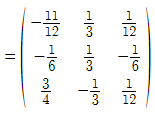### Properties

A few important properties of the inverse matrix are listed below.

• If A is nonsingular, then (A-1)-1  =  A
• If A and B are nonsingular matrices, then AB is nonsingular. Thus, (AB)-1  =  B-1A-1
• If A is nonsingular then (AT)-1  =  (A-1)T
• If A is any matrix and A-1 is its inverse, then AA-1 = A-1A = In, where n is the order of matrices

### Practice Problems

1. Find the inverse of a matrix:
$$\begin{array}{l}A = \begin{bmatrix} 1 & 2 &3 \\ 3 & -2 &1 \\ 4 & 1 & 1 \end{bmatrix}\end{array}$$
2. Obtain the inverse of the matrix using elementary operations:
$$\begin{array}{l} A = \begin{bmatrix} 0 & 1 &2 \\ 1 & 2 &3 \\ 3 & 1 & 1 \end{bmatrix}\end{array}$$

3. Find the inverse of a matrix:
$$\begin{array}{l}\begin{bmatrix} 1 & 2\\ 3 & 4 \end{bmatrix}\end{array}$$

## Frequently Asked Questions – FAQs

Q1

### What is concept inverse of a matrix?

Like numbers, matrices do have reciprocals. In case of matrices, this reciprocal is called inverse matrix. If A is a square matrix and B is its inverse, then the product of two matrices is equal to the unit matrix.
Q2

### How do you find the inverse of a 3×3 matrix?

The steps required to find the inverse of a 3×3 matrix are:
Compute the determinant of the given matrix and check whether the matrix invertible
Calculate the determinant of 2×2 minor matrices
Formulate the matrix of cofactors
Take the transpose of the cofactor matrix to get the adjugate matrix
Finally, divide each term of the adjugate matrix by the determinant
Q3

### Is adjoint and inverse the same?

No, adjoint matrix and inverse matrix are not the same. However, by dividing the each term of the adjugate matrix by the determinant of the original matrix, we get inverse matrix.
Q4

### How to do you know whether the given matrix has inverse?

If the determinant of a given matrix is not equal to 0, then the matrix is invertible and we can find the inverse of such matrix. That means, the given matrix must be non-singular.
Q5

### What are the properties of inverse matrix?

Some of the important properties of inverse matrices are:
The inverse of inverse matrix is equal to the original matrix.
If A and B are invertible matrices, then AB is also invertible. Thus, (AB)^-1 = B^-1A^-1
If A is nonsingular then (A^T)^-1 = (A^-1)^T
The product of a matrix and its inverse and vice versa is always equal to the identity matrix.